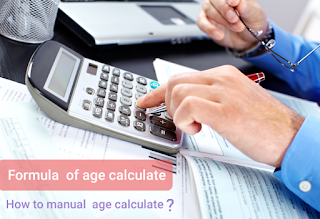### How to calculate date to date or date of birth?Learn the formula.

How to manual date calculate?

Important: here is perfect formula of date calculate. nowadays, many websites and applications using this formula. today i explain some right formulas. let's start
suppose
today date = 25-11-2020 and birth date = 11-06 -2000 `[ used formet: dd-mm-yy ]`

today date = 25-11-2020
birth date = 11-06-2000

### if have birth date smaller from today date so use simple minus method.

Note here today's date months and years larger from birth date month and year.
just like
`       today date :   25 - 11 - 2020`
`       birth date : - 11 - 06 - 2000`
`Use minus method : ----------------`
`      your age =     14 - 05 - 0020 `
There are different rules for the other type of date.

### if have today's month smaller than the birth month so use this method.

for example
` date formats [dd-mm-yyyy]`
today date = 23-06-2020
birth date = 19-11-2000

today month=06
birth month=11;
formula
Months = Total month of year - month of birth + month of today;
Days = today date-birth date;
for date Calculation
days= 23(today date)-19(birth date)
``` days = 23-19 = 4 ;
Total days = 4 ;
```
for the month Calculation md= 12(total month of year)-11(birth month); total month= md+(today month;)
```  12-11 = 1 ;
01+06 = 7 ;
Total month = 7 ;```
for year Calculation today year of date- birth date of month
```  today date = 2020
birth date = 2000
2020-2000 = 20 ;
Total year = 20;
```
Note here today's months smaller from birth month.

### Best way to count the year

Suppose
today date = 28-09-2020
birth date = 19-11-2000
age = today date-birth date ; Total Year= age/1000×24×60×60×365.25 script format
T.Y. = Math.floor(age/1000*24*60*60×365.25);

### A best method for counting days !

date format [mm-dd-yyyy]

Suppose
Total year=23 ;
days counting formula = year/34560000;
or
days counting formula = year/(1000×24×24×60);
` Total days = 23/1000×24×24×60 ;`
total days= 794;
script format
``` Total Days = Math.floor(Total Year/(1000*24*24*60)); ```